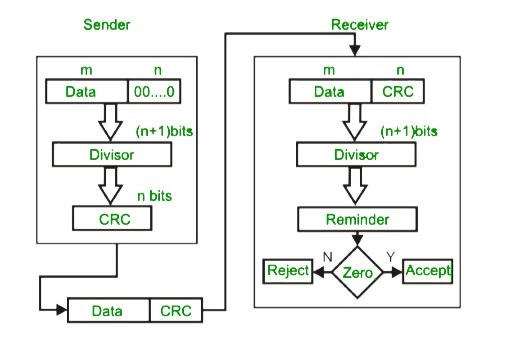# Python Program to Cyclic Redundancy Check

For detecting errors in digital data CRC is used, this is a good technique in detecting the transmission errors. In this technique mainly binary division is applied.

In these technique,cyclic redundancy check bits are present which is a sequence of redundant bits,these bits are appended to the end of data unit so that the resulting data unit becomes exactly divisible by a second which is predetermined binary number.

At the destination side, the incoming data is divided by the same number, if there is no remainder then assumed that data is correct and it’s ready to accept.

A remainder indicates that something happen during transition, data unit has been damaged. So this data unit is not accepted.## Example Code

frompycrc.crclib import *
def main():
#-----------------------------------------------------------------------------
#Sender Side
div = str(input("Input divisor in binary type: "))
#user_dataword = str(raw_input("Input dataword in binary type: "))
userdataword = '1001'
print ("\nSender:")
sen = Sender(bin2dec(userdataword), div)
sen.send()
print ("arg_dataword:", sen.arg_dataword2)
print ("remainder:", sen.remainder2)
print ("codeword:", sen.codeword2)
#-----------------------------------------------------------------------------
#Channel
print ("\nChannel:")
ch = Channel(sen.codeword)
print ("Through to the channel get channel codeword:", dec2bin(ch.ch_codeword))
#-----------------------------------------------------------------------------
print ("syndrome:", rcv.syndrome2)
print ("rx_dataword:", rcv.rx_dataword2)
if __name__ == '__main__':
main()


## Output

Sender
Input dataword in binary type 1010000
arg_dataword:1010000000
remainder: 011
codeword:1010000011